##### Differential Equations For DummiesTo find the area between two curves, you need to come up with an expression for a narrow rectangle that sits on one curve and goes up to another.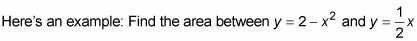from x = 0 to x = 1: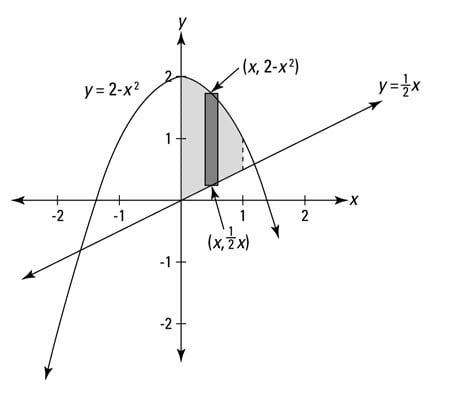To get the height of the representative rectangle in the figure, subtract the y-coordinate of its bottom from the y-coordinate of its top — that’s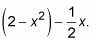Its base is the infinitesimal dx. So, because area equals height times base,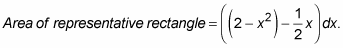Now you just add up the areas of all the rectangles from 0 to 1 by integrating: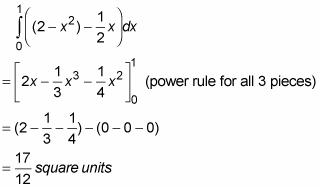Now to make things a little more twisted, in the next problem the curves cross (see the following figure). When this happens, you have to divide the total shaded area into separate regions before integrating. Try this one: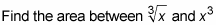from x = 0 to x = 2.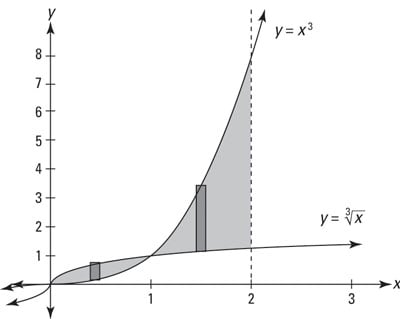1. Determine where the curves cross.

They cross at (1, 1) — what an amazing coincidence! So you’ve got two separate regions — one from 0 to 1 and another from 1 to 2.

2. Figure the area of the region on the left.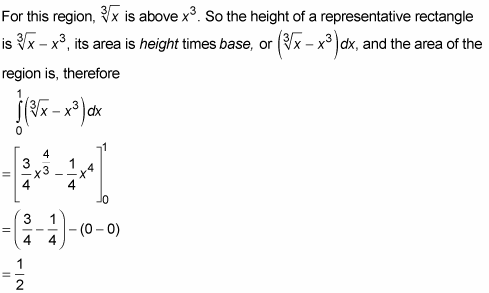3. Figure the area of the region on the right.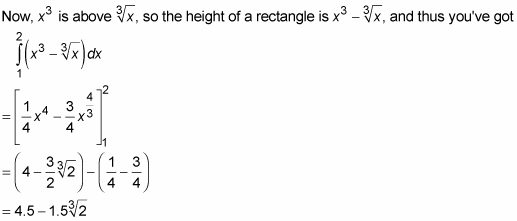4. Add up the areas of the two regions to get the total area.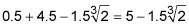≈ 3.11 square units

Note that the height of a representative rectangle is always its top minus its bottom, regardless of whether these numbers are positive or negative. For instance, a rectangle that goes from 20 up to 30 has a height of 30 – 20, or 10; a rectangle that goes from –3 up to 8 has a height of 8 – (–3), or 11; and a rectangle that goes from –15 up to –10 has a height of –10 – (–15), or 5.

If you think about this top-minus-bottom method for figuring the height of a rectangle, you can now see — assuming you didn’t already see it — why the definite integral of a function counts area below the x-axis as negative. For example, consider the following figure.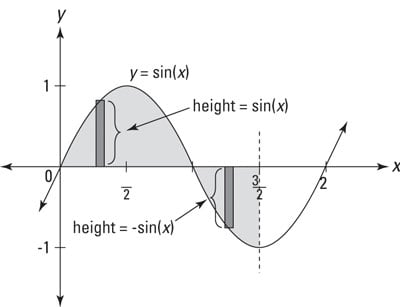What’s the shaded area? Hint: it’s not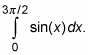If you want the total area of the shaded region shown in the figure, you have to divide the shaded region into two separate pieces like you did in the last problem.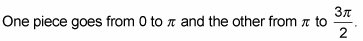For the first piece, from 0 to pi, a representative rectangle has a height equal to the function itself, y = sin (x), because its top is on the function and its bottom is at zero — and of course, anything minus zero is itself. So the area of this first piece is given by the ordinary definite integral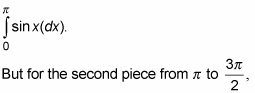the top of a representative rectangle is at zero — recall that the x-axis is the line y = 0 — and its bottom is on y = sin(x), so its height (given, of course, by top minus bottom) is 0 – sin(x), or just –sin(x). So, to get the area of this second piece, you figure the definite integral of the negative of the function,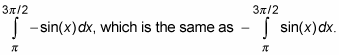Because this negative integral gives you the ordinary, positive area of the piece below the x-axis, the positive definite integral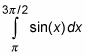gives a negative area. That’s why if you figure the definite integralover the entire span, the piece below the x-axis counts as a negative area, and the answer gives you the net of the area above the x-axis minus the area below the axis — rather than the total shaded area. Clear as mud?